# Optimality, sufficient conditions for

(diff) ← Older revision | Latest revision (diff) | Newer revision → (diff)

Conditions which ensure the optimality of a given solution of a problem of the variational calculus in a chosen class of comparison curves.

Sufficient conditions for optimality of a weak minimum (see ): For a curve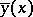to provide a weak minimum for the functional(1)

given the boundary conditions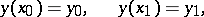it is sufficient that the following conditions are fulfilled.

1) The curvemust be an extremal, i.e. it must satisfy the Euler equation2) Along the curve, including its ends, the strong Legendre condition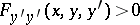must be fulfilled.

3) The curvemust satisfy the strong Jacobi condition, which requires that the solution of the Jacobi equation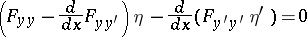(2)

with initial conditionsmust not vanish at the points of the right-closed interval.

The coefficients of the Jacobi equation (2), which is a linear differential equation of the second order, are calculated along the extremaland represent known functions in.

For a strong minimum it is sufficient that the following extra condition be fulfilled, next to those mentioned above.

4) There exists a neighbourhood of the curvesuch that at each point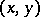of it, and for any, the inequality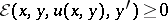(3)

hold, whereis the Weierstrass function, whileis the slope of the field of extremals surrounding.

At the extremal, condition (3) takes the form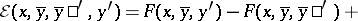(4)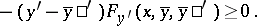Condition (4) is necessary for a strong minimum; it is called the Weierstrass necessary condition (cf. Weierstrass conditions (for a variational extremum)). Thus, unlike the sufficient conditions for a weak minimum, which require certain strong necessary conditions to be fulfilled at the points of the extremal, the sufficient conditions of a strong minimum require that the Weierstrass necessary condition be fulfilled in a neighbourhood of the extremal. In general, it is impossible to weaken the formulation of the sufficient conditions for a strong minimum once the requirement that the Weierstrass condition be fulfilled in a neighbourhood of the extremal has been replaced by the strong Weierstrass condition (condition (4) with strict inequality) at the points of the extremal (see ).

For non-classical variational problems, examined in the mathematical theory of optimal control (cf. Optimal control, mathematical theory of), several approaches to the establishment of sufficient conditions for optimality of an absolute extremum exist.

Let a problem of optimal control be posed in which the minimum of the functional(5)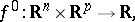has to be determined, given the conditions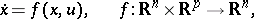(6)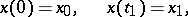(7)(8)

whereis a given closed set in-dimensional space.

Using the method of dynamic programming , sufficient conditions for optimality are formulated in the following way. For a control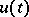to be an optimal control in the problem (5)–(8), it is sufficient that:

a) there exists a continuous functionwhich has continuous partial derivatives for allwith the possible exception of a certain piecewise-smooth set of dimension less than, which vanishes at the end-point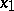,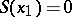, and which satisfies the Bellman equation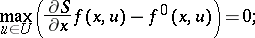(9)

b)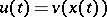if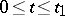, whereis a synthesizing function (cf. also Optimal synthesis control), which can be defined using the Bellman equation:In reality, when using the method of dynamic programming, a stronger result is obtained: Sufficient conditions for optimality for a set of different controls which transfer a phase point from an arbitrary initial state to a given final state.

In the more general case of a non-autonomous system, i.e. the integrand and the vector-function of right-hand sides also depend on the time, the functionwill depend onand a term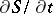must be added to the left-hand side of equation (9). There is a proof (see ) which assumes the condition of continuous differentiability of the functionfor all, which is very restrictive and is not fulfilled in most problems although it is usually supposed.

Sufficient conditions for optimality can be constructed on the basis of the Pontryagin maximum principle. If in a regionof the phase space a regular synthesis is realized (cf. Optimal synthesis control), then all trajectories obtained using the maximum principle when constructing the regular synthesis are optimal in.

The definition of a regular synthesis, although rather cumbersome, does not essentially impose any particular restrictions on the problem (5)–(8).

There is another approach to the construction of sufficient conditions for optimality (see ). Let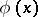be a continuous function with continuous partial derivatives for all admissiblebelonging to a given region, and let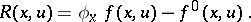(10)

For the pairto establish an absolute minimum in the problem (5)–(8), it is sufficient that there exists a functionsuch that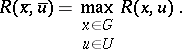(11)

Corresponding variations of the above-mentioned formulation of the sufficient conditions for optimality are permissible for the more general cases of non-autonomous systems, problems with Mayer- and Bolza-type functions (see Bolza problem), as well as for optimal sliding regimes (see Optimal sliding regime and ).

Research has been carried out into variational problems with functionals in the form of multiple integrals and with differential constraints in the form of partial differential equations, in which functions of several variables occur (see ).

How to Cite This Entry:
Optimality, sufficient conditions for. Encyclopedia of Mathematics. URL: http://encyclopediaofmath.org/index.php?title=Optimality,_sufficient_conditions_for&oldid=13932
This article was adapted from an original article by I.B. Vapnyarskii (originator), which appeared in Encyclopedia of Mathematics - ISBN 1402006098. See original article